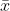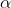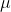##### Question

In: Statistics and Probability

# The mean number of flying times for pilots at Southwest Airlines is X ̅ = 80...

The mean number of flying times for pilots at Southwest Airlines is X ̅ = 80 hours per month. Assume that this mean was based on actual flying times for a sample of n = 25 Southwest Airlines pilots and that the sample standard deviation was S = 8.5 hours.

a.Construct and interpret a 95% confidence interval estimate of the population mean flying time for the Southwest Airlines pilots.

b.What is the margin of error in part a?

## Solutions

##### Expert Solution

Solution :

Given that,= 80

s = 8.5

n = 25

Degrees of freedom = df = n - 1 = 25 - 1 = 24

a ) At 95% confidence level the t is ,= 1 - 95% = 1 - 0.95 = 0.05/ 2 = 0.05 / 2 = 0.025

t/2,df = t0.025,24 =2.797

b ) Margin of error = E = t/2,df * (s /n)

= 2.797 * (8.5/ 25)

= 4.75

Margin of error = 4.75

The 99% confidence interval estimate of the population mean is,- E <<+ E

80 - 4.75 << 80 + 4.75

75.25 << 84.75

(75.25, 84.75 )

## Related Solutions

##### The mean number of flying times for pilots at Southwest Airlines is X ̅ = 80...
The mean number of flying times for pilots at Southwest Airlines is X ̅ = 80 hours per month. Assume that this mean was based on actual flying times for a sample of n = 25 Southwest Airlines pilots and that the sample standard deviation was S = 8.5 hours. a.Construct and interpret a 95% confidence interval estimate of the population mean flying time for the Southwest Airlines pilots. b.What is the margin of error in part a?
##### Research case studies on Southwest Airlines. Then answer the following question. 1. What is the Southwest...
Research case studies on Southwest Airlines. Then answer the following question. 1. What is the Southwest business model and why is it so effective?
##### Use Southwest Airlines’ 2016 financial statement information,below to answer the following:Calculate Southwest Airlines’return...
Use Southwest Airlines' 2016 financial statement information, below to answer the following:Calculate Southwest Airlines’ return on assets (ROA) for the year ending December 31, 2016.Disaggregate Southwest Airlines' ROA into profit margin (PM) and asset turnover (AT). Explain what each ratio measures.($millions)Total operating revenues$20,425Net income2,244Total assets, beginning of year21,312Total assets, end of year23,286Equity, end of year8,441
##### A simple random sample of 12 reaction times of helicopter pilots is selected. The reaction times...
A simple random sample of 12 reaction times of helicopter pilots is selected. The reaction times have a normal distribution. The sample mean is 1.67 sec with a standard deviation of 0.16 sec. Construct a 90% confidence interval for the population standard deviation. 1. 0.11 < σ < 0.23 2. 0.01 < σ < 0.05 3. 0.12 < σ < 0.25 4. 0.13 < σ < 0.22
##### Delta Airlines is trying to determine if pilots are deliberately slowing down during a labor dispute....
Delta Airlines is trying to determine if pilots are deliberately slowing down during a labor dispute. They know that their all their flights have a mean late time of 12.8 minutes with a standard deviation of 5.8 minutes. They took a random sample of 36 flights during the dispute and found they were 15.1 minutes late on average. Using a significance level of 0.05, is there any evidence to back their claim that the pilots are slowing down?
##### Delta Airlines is trying to determine if pilots are deliberately slowing down during a labor dispute....
Delta Airlines is trying to determine if pilots are deliberately slowing down during a labor dispute. They know that their all their flights have a mean late time of 12.8 minutes with a standard deviation of 5.8 minutes. They took a random sample of 36 flights during the dispute and found they were 15.1 minutes late on average. Using a significance level of 0.05, is there any evidence to back their claim that the pilots are slowing down?
##### Delta Airlines is trying to determine if pilots are deliberately slowing down during a labor dispute....
Delta Airlines is trying to determine if pilots are deliberately slowing down during a labor dispute. They know that their all their flights have a mean late time of 12.8 minutes with a standard deviation of 4.8 minutes. They took a random sample of 31 flights during the dispute and found they were 14.1 minutes late on average. Using a significance level of 0.05, is there any evidence to back the pilots claim that they are not slowing down?
##### recent report for a regional airline reported that the mean number of hours of flying time...
recent report for a regional airline reported that the mean number of hours of flying time for its pilots is 66 hours per month. This mean was based on actual flying times for a sample of 45 pilots and the sample standard deviation was 9 hours. 2. Calculate a 99% confidence interval estimate of the population mean flying time for the pilots. Round your result to 4 decimal places. 3.Using the information given, what is the smallest sample size necessary...
##### Southwest Airlines provides scheduled air transportation services in the United States. Like many airlines, South-west leases...
Southwest Airlines provides scheduled air transportation services in the United States. Like many airlines, South-west leases many of its planes from Boeing Company. In its long-term debt disclosure note included in the financial statements for the year ended December 31, 2015, the company listed $324 million in lease obligations. The existing leases had an approximate ten-year remaining life and future lease payments average approximately$45 million per year. Required: 1. Determine the effective interest rate the company used to determine...